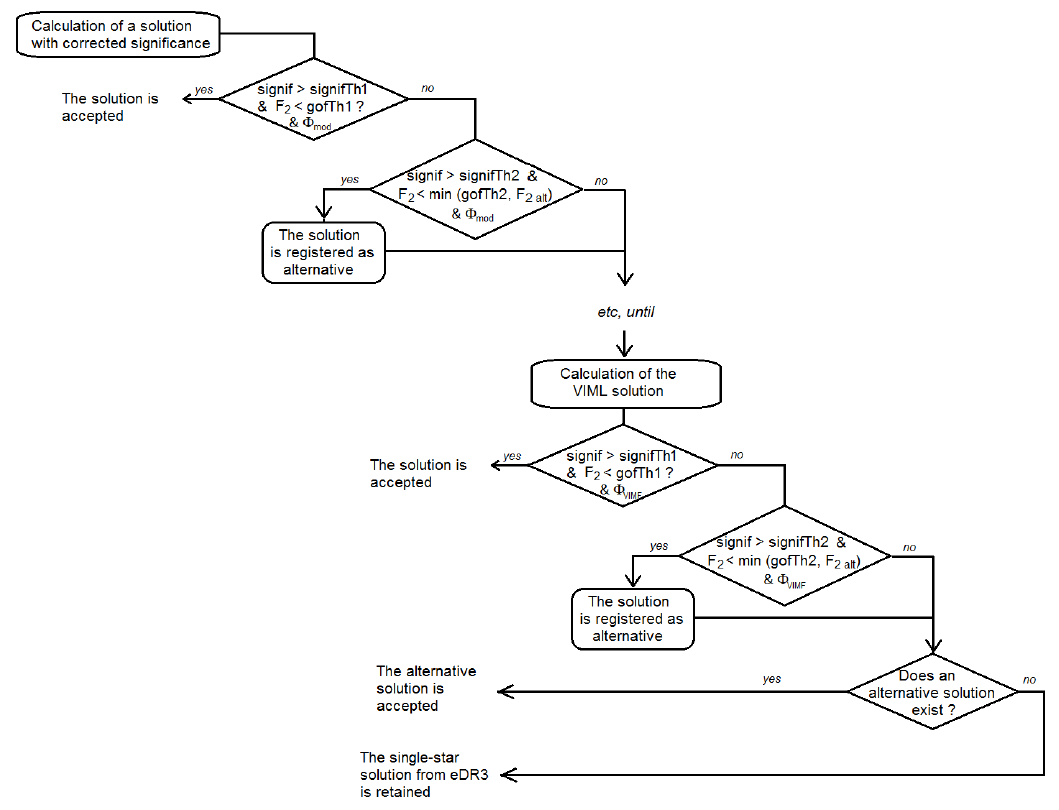# 7.2.3 Acceptance of solutions

## Basic criteria: goodness-of-fit and significance

The acceptance of the solutions was based on several criteria, depending on the model. However, in the main processing, only two quantities were considered: the estimator of the goodness_of_fit $F2$, and the significance.

We used the goodness-of-fit defined in Stuart and Ord (1994) as:

 $F2=\sqrt{\frac{9\nu}{2}}\left[\left(\frac{\chi^{2}}{\nu}\right)^{1/3}+\frac{2}% {9\nu}-1\right]$ (7.3)

where $\nu$ is the number of degrees of freedom, and $\chi^{2}$ is the sum of the squared normalised residuals. This estimator was already the basis for the acceptance of the solutions of the Hipparcos programme (see ESA 1997, volume 1, Section 2.1). When the model applied is adequate and when the estimations of the measurement errors are right, $F2$ should follow the normal distribution ${\cal N}(0,1)$.

The second quantity taken into account is the significance. Like $F2$, the significance was also used in the preparation of the Hipparcos catalogue, to decide whether to select a constant or variable acceleration solution. When comparing two models that differ by the addition of a vector, significance is the norm of that vector divided by its uncertainty. For Gaia, this concept has been extended by defining, for each model, a significance that characterises it. Significance plays a primary role in the selection of solutions for Gaia DR3: as the uncertainties of the epoch astrometric measurements are generally very underestimated, a selection based on $F2$ alone would mean rejecting almost all solutions. It was therefore decided to use $F2$ to calculate the correction that must be applied to the measurement uncertainties in order to have a zero $F2$. It follows from Equation 7.3 that the measurement uncertainties should be multiplied by the coefficient:

 $c=\sqrt{\frac{\chi^{2}}{\nu\left(1-\frac{2}{9\nu}\right)^{3}}}$ (7.4)

which is approximately equal to $\sqrt{\chi^{2}/\nu}$, since the number of CCD transits is very large. In practice, this correction was applied to the uncertainties of the solution parameters, and it was taken into account in any significance estimation.

## Rejection of outliers in the calculation of solutions of linear models

In addition to the intercomparison filtering of the CCD transits performed in the preprocessing, Section 7.2.2, transits with a normalised residual greater than 5 could also be rejected. This operation was subject to several conditions:

• The solution must come from a linear model: single-star model or acceleration model. The VIMF model is not affected because of the inclusion of model uncertainty (see Section 7.2.6).

• The uncorrected $\chi^{2}$ must be larger than 1.41 times the number of transits still considered. As the number of transits is large, it comes from Equation 7.3 that this condition is roughly equivalent to $F2>0.26\sqrt{\nu}$.

• The total amount of outliers rejected in this process is limited to 5 % of the initial number of transits.

The calculation of the solution, followed by this process of rejecting transits, is repeated until the rejection conditions are no longer satisfied.

## Basic acceptance processFigure 7.3: Schematic diagram of the solution acceptance process. In practice, the thresholds were signifTh1=12, GoFTh1=25, signifTh2=5 and GoFTh2=1 000. Φmod is an additional filter depending on the model.

The process of deciding whether to accept a solution is illustrated in the diagram in Figure 7.3. The acceptance of a solution is based on a filter specific to each model, noted $\Phi_{\mathrm{mod}}$, and on two pairs of thresholds each applied to $F2$ and significance. The first two thresholds, labelled “1”, are used to decide whether to accept a solution immediately. In this case, no other model will be tried. The second thresholds are less severe, and allow to say if a solution could be accepted as an alternative, for lack of better. The alternative solution with the lowest $F2$ will finally be accepted at the end of the calculation. The calculation of successive solutions was done by applying immediate acceptance thresholds of 25 and 12 for $F2$ and significance, respectively. Alternative solutions were taken into account when their significance was greater than 5 and $F2$ was less than 1000.

It should be noted that the schematic diagram presented here does not apply to the choice of acceleration solutions, for which the process has been modified to favour the variable acceleration solution (see Section 7.2.4).

Note also that this cascading process favours the models that are tried first. Thus, many binaries that could have had an orbital solution remained on an acceleration solution. This choice was made in order to favour the quality of the orbital solutions, assuming that they would be questionable for stars that also accept an acceleration solution.